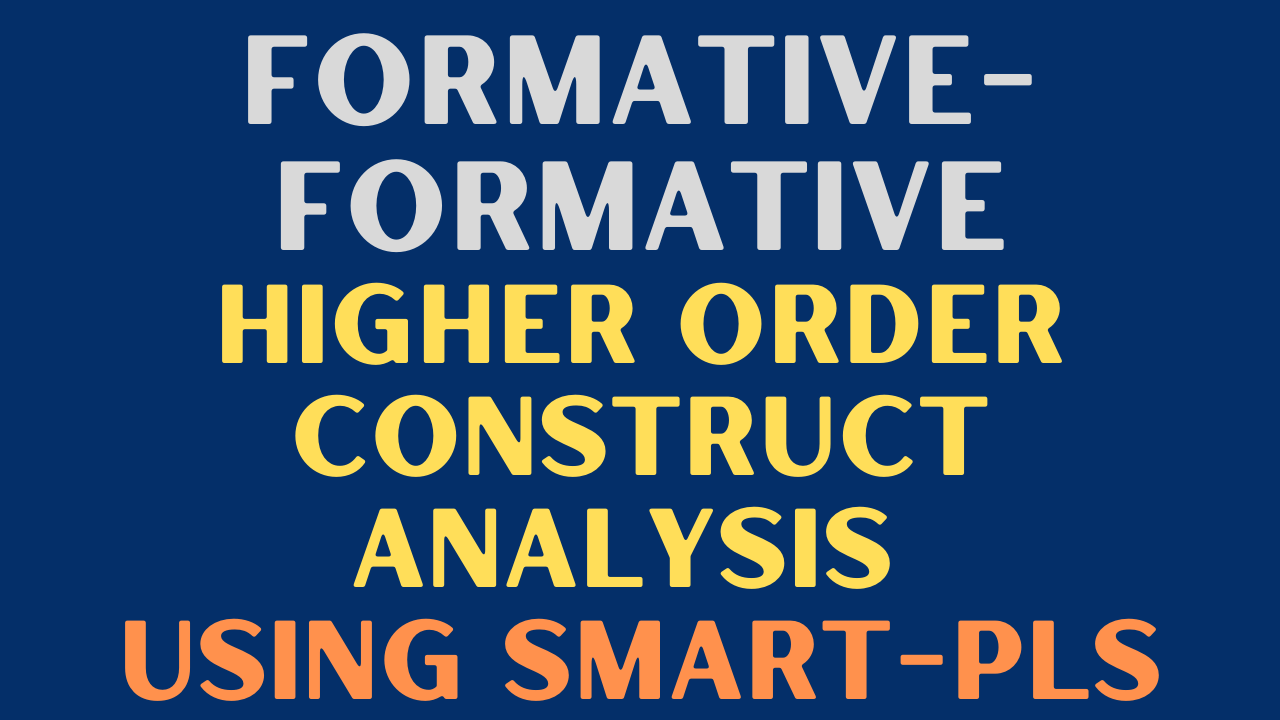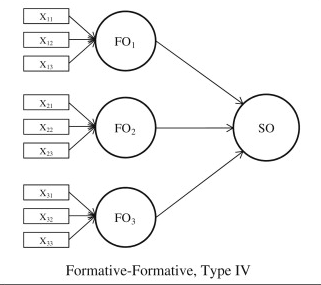# Formative-Formative Higher Order Construct Analysis using SmartPLS### Formative-Formative Higher Order Construct Analysis using SmartPLS

In CB-SEM, Loadings, Model Fit, and Modification Indices are critical to model identification. This post will discuss in detail all these concept.

## Analyzing Formative-Formative Higher Order Constrcut in SmartPLS

A Formative-Formative model is one where the first order has formative indicators while in the second-order, the dimensions are also formative. Here is how it looks## Two Stage Approach

In order to analyze the formative-formative indicators, this tutorial will use the disjoint two stage approach. To know more about the approaches, Click here

## Disjoint Two Stage Approach

• The disjoint two-stage approach considers only the lower-order components of the higher-order construct (i.e., without the higher-order component) in the path model at Stage 1.
• These are directly linked to all other constructs that the higher-order construct is theoretically related to.
• To execute the disjoint two-stage approach, researchers then need to save the construct scores (LVS), but only those of the lower order components.
• In stage two, these scores are then used to measure the higher-order construct.

## Step 1: Validation of LOCs

• The Step 1 is the validation of the LO formative constructs. The procedure used is the same as we use for a Formative construct as explained in the tutorial here.
• Note: If there are other constructs in the study that are reflective, they will be validated as per the guidelines of reflective constructs (Construct Reliability, Convergent Validity, and Discriminant Validity)
• The next step after validation is to generate Latent Variable Scores (LVS).
• Save the LVS score in your datasheet, and import the datasheet again into the SmartPLS.

## Step 2: Validation of HOC

• Once the new datasheet is imported, add LOCs as indicators to the HO formative construct.
• Add other LOCs or HOCs in the model as required.
• Validation of the HO formative construct, is the same as we did in step 1. Further steps are explained in the tutorial here.
• Next, after validation, assess the model for significance of relationships.